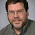## Thursday, November 19, 2015

### A Silly String Theorem

First a note on a serious theorem: Babai has posted a video (mp4, 1h 40 m, 653MB) of his first talk on his Graph Isomorphism algorithm.

I was giving a talk on the Kleene star operator (don't ask) and came across this cute little problem. Say a language L commutes if for all u,v in L, uv=vu.

Problem:
Show that L commutes if and only if L is a subset of w* for some fixed string w.

Here w* is the set of strings consisting of zero or more concatenations of w with itself. The if case is easy, but I found the other direction pretty tricky and came up with an ugly proof. I found a cleaner proof in Seymour Ginsburg's 1966 textbook The Mathematical Theory of Context Free Languages which I present here.

If u=wi and v=wj then uv=vu=wi+j.

Trivial if L contains at most one string. Assume L contains at least two strings.

Proof by induction on the length of the shortest non-empty string v in L.

Base case: |v|=1
Suppose there is an x in L with x not in v*. Then x = vibz for b in Σ-{v}. Then the i+1st character of xv is b and the i+1st character of vx is v, contradicting xv=vx. So we can let w=v.

Inductive case: |v|=k>1.

Lemma 1: Let y=vru be in L (u might be empty). Then uv=vu.
Proof: Since yv=vy we have vruv=vvru=vrvu. So vu=uv.

Let x in L be a string not in v* (if no such x exists we can let w=v). Pick j maximum such that x=vjz with z not the empty string. By Lemma 1 we have zv=vz. Note |z| < |v| otherwise v is a prefix of z, contradicting the maximality of j.

Lemma 2: For all y in L we have yz=zy.
Proof: As before let y = vru. By Lemma 1, uv=vu
Since yx=xy we have vruvjz = vjzvru
vruvjz = vruzvj by swapping v's and z's.
vjzvru = zvruvj by swapping v's and z's, and v's and u's.
So we have  vruzvj = zvruvj
or yzvj = zyuj and thus yz=zy.

By Lemma 2, the set L∪{z} commutes. Since 0 < |z| < |v| by induction L∪{z} is a subset of w* for some w so L is a subset of w*.

1.Lyndon's theorem: If xy = yx then x and y are both powers of the same string.

If y = s^i = t^k then there is a u such that s = u^m and t = u^n.

2.Yeah, as anonymous is saying, you're basically reproving a classic theorem from combinatorics on words known as the Lyndon-Schutzenberger theorem. Once you know this basic result, the proof follows almost immediately. See my book, "A Second Course in Formal Languages and Automata Theory", Theorem 2.3.3.

3.And here I was thinking I'd beat Jeffrey Shallit to pointing out that the result is in his book.

4.this is like euclid's gcd algorithm